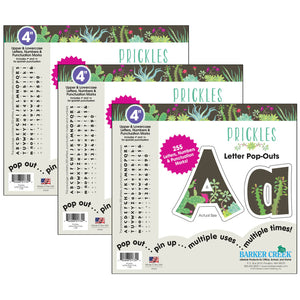Barker Creek

# (3 Pk) 4in Letter Popouts Prickles

Regular price \$58.75 \$0.00
Tax included.
Brighten up any space with Prickles 4" Letter Pop-Outs. Super chic and easy to read, they are perfect for your next project, presentation, report, poster, or display! The designs feature a chocolate brown color with various cacti and succulents in beautiful blues and greens along with lovely magenta flowers. Each set includes a well-researched assortment of 255 letters, numbers, and punctuation marks (including Spanish). Sold as 3 sets for a total of 765 pieces. Each individual set includes: A = 3, B = 2, C = 3, D = 3, E = 3, F = 3, G = 3, H = 3, I = 3, J = 2, K = 2, L = 3, M = 3, N = 3, O = 3, P = 3, Q = 2, R = 3, S = 3, T = 3, U = 3, V = 2, W = 3, X = 2, Y = 3, Z = 2, a = 6, b = 3, c = 4, d = 4, e = 6, f = 4, g = 4, h = 4, i = 6, j = 3, k = 3, l = 4, m = 4, n = 4, o = 4, p = 4, q = 3, r = 4, s = 4, t = 4, u = 4, v = 3, w = 4, x = 3, y = 4, z = 3, ? = 2, ! = 2, ¢=2, \$ = 2, & = 2, . = 18, ' = 17, - = 3, " = 4, ~ = 4 ` = 5 and 2 each of numbers 0-9.

Key Features :

Brighten up any space with Petals 4" Letter Pop-OutsSuper chic and easy to read, they are perfect for your next project, presentation, report, poster, or displayThe designs feature a bold navy color with various flowers in magenta, purple, white and green255 pieces per set (letters, numbers, and punctuation marks (including Spanish)), sold as 3 sets, 765 pieces total